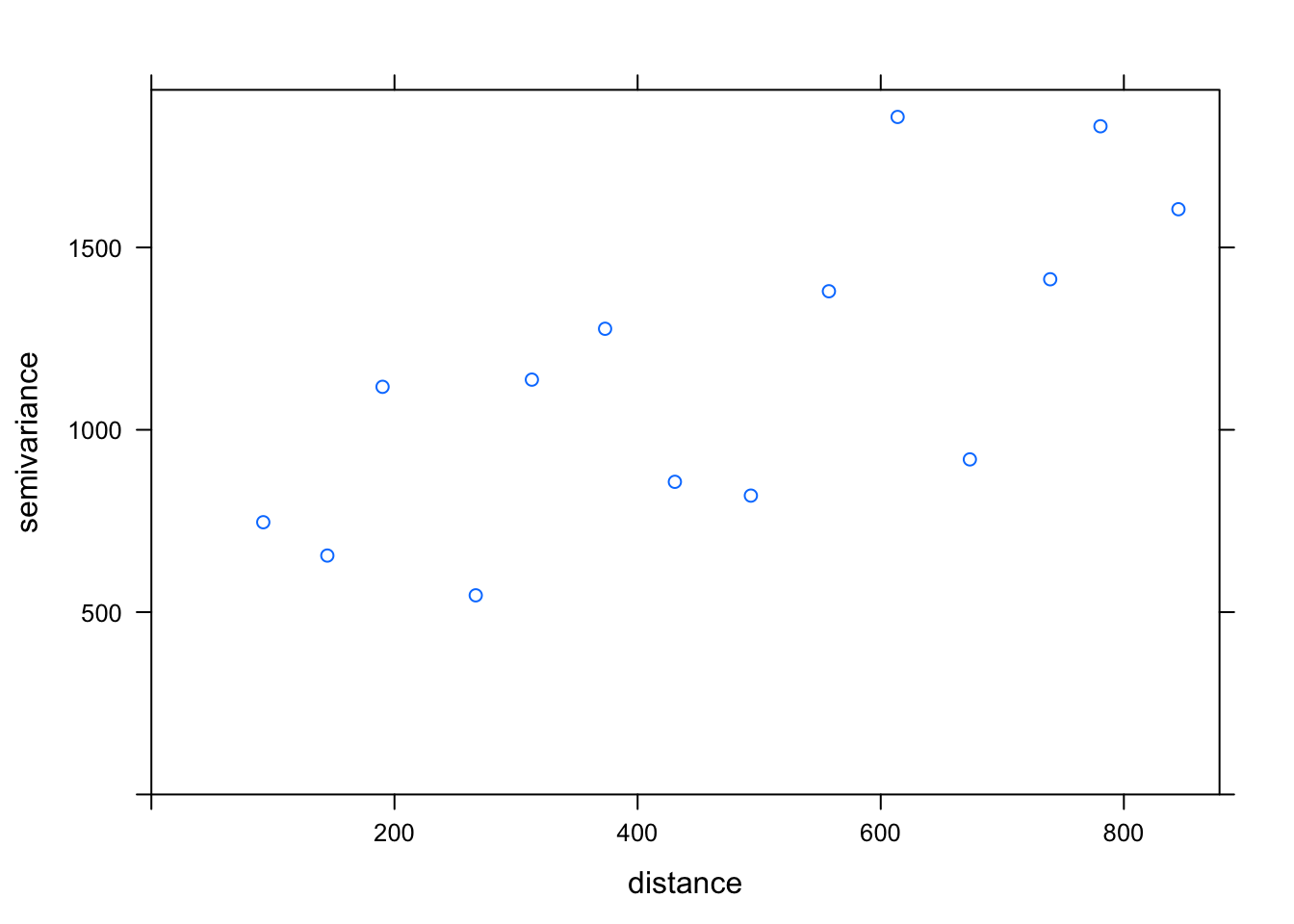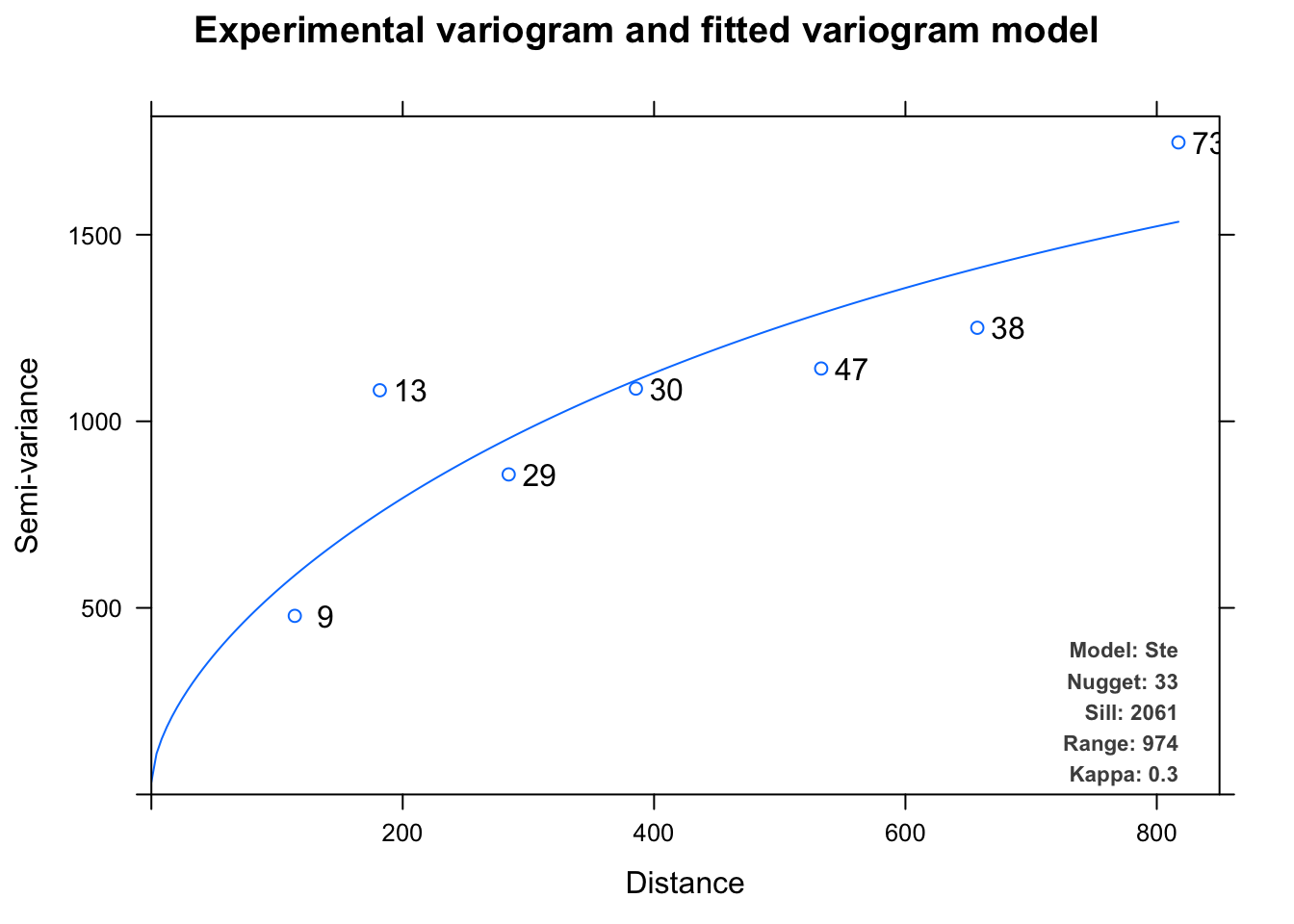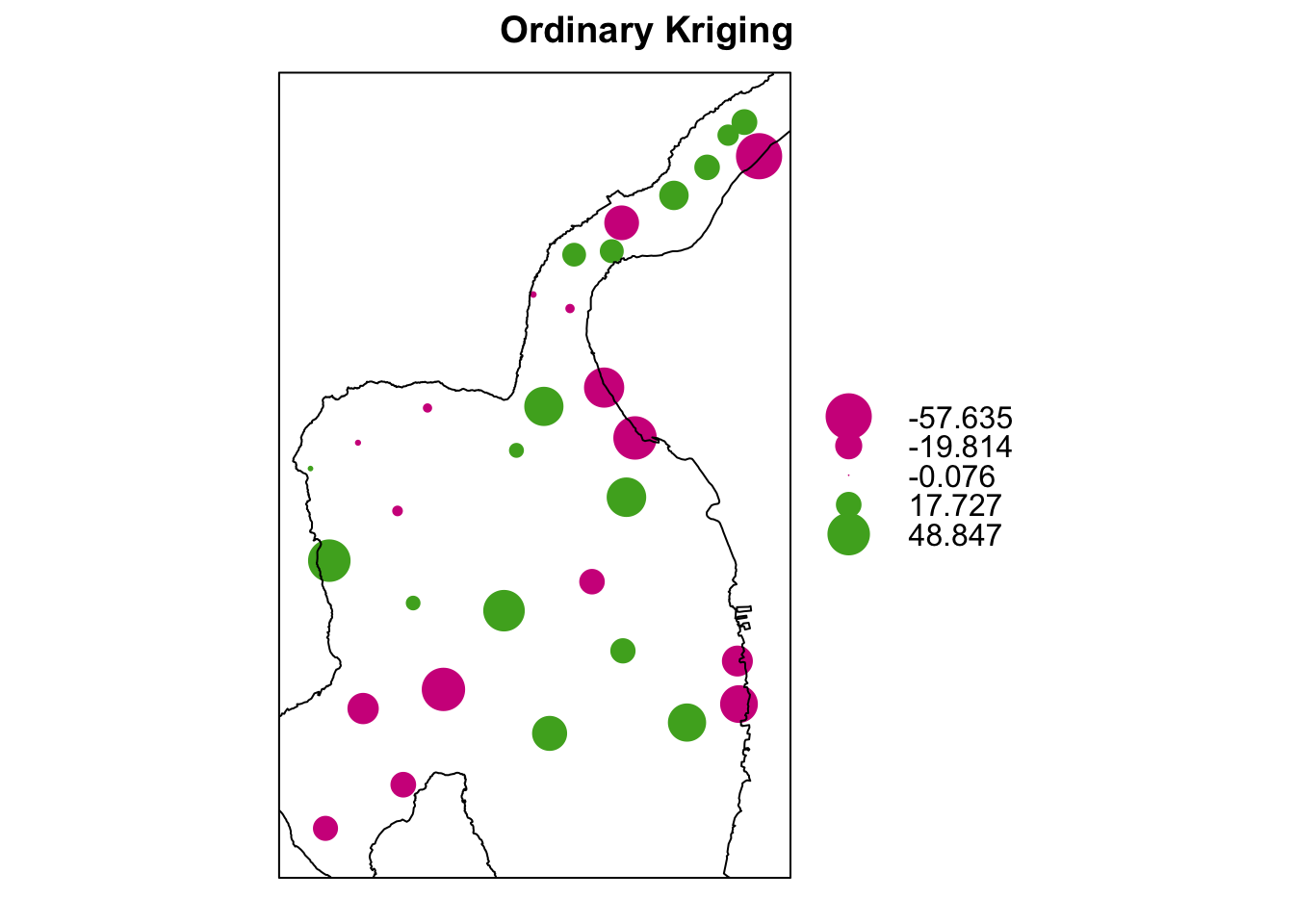In this section we apply ordinary kriging to predict the spatial distribution of zinc in Lake Rangsdorf based on sediment samples taken during a field survey in 2017.

#### Data preparation

We start with our data set, `lake.data.sf`, a object of class `sf`.

``````library(sf)
str(lake.data.sf)``````
``````## Classes 'sf' and 'data.frame':   32 obs. of  23 variables:
##  \$ ID             : Factor w/ 32 levels "10a","11a","12a",..: 1 2 3 4 5 6 7 8 9 10 ...
##  \$ depth_m        : num  0.68 0.66 1.93 3.98 4.95 0.93 3.92 8.4 5.15 4 ...
##  \$ visual.depth.cm: int  34 28 15 15 18 20 18 18 15 15 ...
##  \$ elec.cond.muS  : int  305 274 680 825 903 416 1060 1278 795 910 ...
##  \$ pH             : num  7.19 7.33 7.25 7.22 7.05 7.31 6.82 7.02 6.99 7.01 ...
##  \$ LOI550         : num  1.04 1.27 27.32 36.93 38.33 ...
##  \$ LOI990         : num  0.2 0.19 17.46 18.25 15.5 ...
##  \$ LOI            : num  98.5 98.3 33 21.6 26.4 ...
##  \$ C              : num  0.85 0.93 19 24.4 23.7 1.79 24.7 23.1 22.8 25.4 ...
##  \$ N              : num  NA NA 1.18 1.85 1.89 NA 1.94 1.82 1.75 2.09 ...
##  \$ Ca.mg.g        : num  9.83 3.78 172.12 182.74 193.42 ...
##  \$ Fe.mg.g        : num  0.52 0.42 4.73 8.58 10.12 ...
##  \$ K.mg.g         : num  0.22 0.13 0.34 0.43 0.5 0.14 0.55 0.64 0.41 0.52 ...
##  \$ Pb.mug.g       : num  1.37 1.81 18.49 34.19 31 ...
##  \$ Mg.mg.g        : num  0.15 0.13 1.41 1.47 1.42 0.2 1.46 1.45 1.35 1.44 ...
##  \$ Mn.mug.g       : num  15.4 12 481.6 454.4 546.7 ...
##  \$ Na.mg.g        : num  0.26 0.22 0.54 0.7 0.88 0.32 0.74 0.72 0.63 0.82 ...
##  \$ PO4.mg.g       : num  0.3 0.37 4.83 4.93 5.31 1.18 5.34 5.97 4.83 5.51 ...
##  \$ S.mg.g         : num  0.77 0.43 8.24 17.18 20.03 ...
##  \$ Sr.mug.g       : num  7.87 6.77 238.28 261.05 245.53 ...
##  \$ Zi.mug.g       : num  11.5 17.4 84.2 94.9 98.5 ...
##  \$ geometry       :sfc_POINT of length 32; first list element:  'XY' int  391829 5794052
##  \$ dist           : num  27.54 6.85 10.09 68.47 93.63 ...
##  - attr(*, "sf_column")= chr "geometry"
##  - attr(*, "agr")= Factor w/ 3 levels "constant","aggregate",..: NA NA NA NA NA NA NA NA NA NA ...
##   ..- attr(*, "names")= chr  "ID" "depth_m" "visual.depth.cm" "elec.cond.muS" ...``````

Here we are only interested in the `Zi.mug.g` variable. Hence we subset the data set. Note that we do not have to specify the `geometry` column to be included. After subsetting to one variable the object is still of class `sf`.

``````lake.zinc <- lake.data.sf[,'Zi.mug.g']
str(lake.zinc)``````
``````## Classes 'sf' and 'data.frame':   32 obs. of  2 variables:
##  \$ Zi.mug.g: num  11.5 17.4 84.2 94.9 98.5 ...
##  \$ geometry:sfc_POINT of length 32; first list element:  'XY' int  391829 5794052
##  - attr(*, "sf_column")= chr "geometry"
##  - attr(*, "agr")= Factor w/ 3 levels "constant","aggregate",..: NA
##   ..- attr(*, "names")= chr "Zi.mug.g"``````

For future usage we cast the `sf` object into a spatial object of class `sp`.

``````library(sp)
lake.zinc.sp <- as(lake.zinc, 'Spatial')``````

#### Sample variogram

For the sake of reproducibility we first define a `formula` object, that defines the dependent variable as a linear model of independent variable.

``````# formula object
f <- formula(Zi.mug.g~1)``````

Now we build the sample variogram using the `variogram()` function.

``````library(gstat)
ev <- variogram(f, lake.zinc.sp)
plot(ev)``````#### Variogram modelling

We build a variogram model using the `autofitVariogram()` function from the `automap` package, and plot it.

``````library(automap)
vm = autofitVariogram(f, lake.zinc.sp)
plot(vm)``````#### Model evaluation

We evaluate the variogram model by applying leave-one-out cross-validation (LOOCV), using the `krige.cv()` command.

``````OK.LOOCV <- krige.cv(formula = f,
locations = lake.zinc.sp,
model = vm\$var_model)``````

We plot the model residuals using the `bubble()` function. We add the lake shore line to the plot. Note that we use the `::` notation to make sure we apply the `layer()` function from the correct package namespace.

``````bubble(OK.LOOCV,
"residual",
main = "Ordinary Kriging") +
latticeExtra::layer(sp.polygons(as(lake, 'Spatial')))``````#### Spatial prediction

Finally, we may use our model to predict the zinc concentration in Lake Rangsdorf. Note that we could apply the `krige()` function, a wrapper method around the `gstat()` and `predict()` functions. This time however, we use another handy function, the `interpolate` function from the `raster` package.

First we build a `gstat` object using the `gstat()` function.

``````g = gstat(formula = f,
data = lake.zinc.sp,
model = vm\$var_model)``````

The we use the `interpolate` function, which accepts a `Raster` object and a model object. Note that we first cast the the variable `lake.interpolation`, an object of class `SpatialPixelsDataFrame` to a `RasterLayer` object by applying the `raster` function.

``````library(raster)
zinc = interpolate(object = raster(lake.interpolation),
model = g)``````
``## [using ordinary kriging]``

The resulting `RasterLayer` can be processed like any other raster data set. Hence we mask out the area outside the lake shoreline. Then we plot it using the generic `plot()` function. However, please note that we could as well have used all of the functionality provided by the `raster` and `rasterVis` package for advanced visualizations.

``````# masking